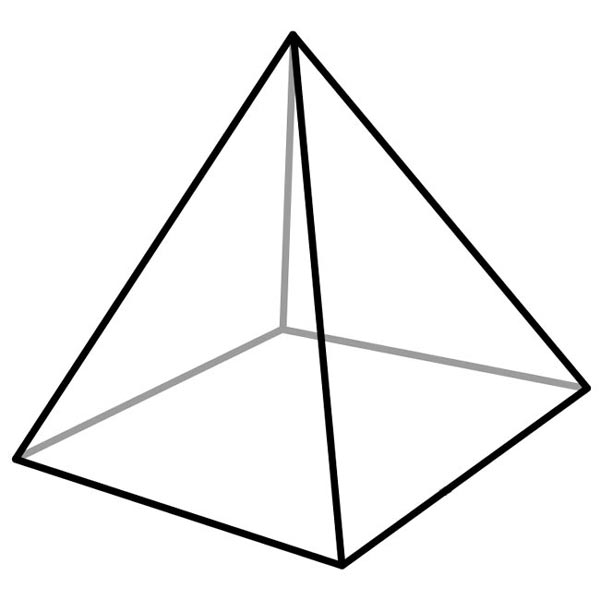# End Of Year Puzzling Practice Swyk

20 Questions | Total Attempts: 227Settings• 1.
How many faces does a square pyramid have?
• A.

4

• B.

5

• C.

6

• D.

7

• 2.
How many edges does a rectangular prism have?
• A.

4

• B.

8

• C.

12

• D.

20

• 3.
Find the area of this parallelogram.  (Area for a parallelogram = base x height.)
• A.

105 cm²

• B.

35 cm²

• C.

75 cm²

• D.

60 cm²

• E.

55

• F.

90

• 4.
4,125 + 2,698 =
• A.

6,823

• B.

7,514

• C.

6,478

• D.

5,784

• 5.
84.28 + 125.31 =
• A.

210.41

• B.

300.45

• C.

60.45

• D.

209.59

• E.

210.88

• 6.
511 - 389 =
• A.

987

• B.

132

• C.

122

• D.

141

• 7.
What is the mode for this set of data? [3, 10, 4, 2, 4, 6, 4, 8, 10, 3]
• A.

4

• B.

10

• C.

6

• D.

5

• 8.
What is the range for this set of data? [3, 10, 4, 2, 4, 6, 4, 8, 10, 3]
• A.

10

• B.

8

• C.

12

• D.

5

• 9.
8 x 514 =
• A.

5,213

• B.

4,232

• C.

4,112

• D.

4,141

• 10.
547 x 124 =
• A.

67,452

• B.

67,145

• C.

54,125

• D.

67,828

• E.

67,448

• 11.
486 / 54 =
• A.

24

• B.

9

• C.

8

• D.

7 R3

• 12.
45 = m - 24
• A.

12

• B.

32

• C.

70

• D.

69

• 13.
A spinner is divided into 5 equal sections.  If you spin the spinner 100 times, how many times do you expect to land on Orange?
• A.

100

• B.

1

• C.

20

• D.

50

• E.

25

• 14.
Jake has 28 cupcakes.  Jake and his 6 friends share them equally.  How many cupcakes does each person get?
• A.

2

• B.

14

• C.

4 R 4

• D.

4

• E.

5

• F.

3

• 15.
A cat sleeps 15 hours a day.  How many hours does a cat sleep during a week?
• A.

105 hrs

• B.

75 hrs

• C.

45 hrs

• D.

60 hrs

• 16.
(54 - 10) x 2 =
• A.

34

• B.

88

• C.

145

• D.

20

• 17.
41 - (9 + 4) * 2 + 1 =
• A.

46

• B.

27

• C.

23

• D.

16

Related TopicsBack to top